Courses

Integer Answer Type Questions: Heat & Thermodynamics | JEE Advanced Notes | Study Physics 35 Years JEE Main & Advanced Past year Papers - JEE

JEE: Integer Answer Type Questions: Heat & Thermodynamics | JEE Advanced Notes | Study Physics 35 Years JEE Main & Advanced Past year Papers - JEE

The document Integer Answer Type Questions: Heat & Thermodynamics | JEE Advanced Notes | Study Physics 35 Years JEE Main & Advanced Past year Papers - JEE is a part of the JEE Course Physics 35 Years JEE Main & Advanced Past year Papers.
All you need of JEE at this link: JEE

Q.1.A metal rod AB of length 10x has its one end A in ice at 0.°C, and the other end B in water at 100 °C. If a point P onthe rod is maintained at 400 °C, then it is found that equal amounts of water and ice evaporate and melt per unit time. The latent heat of evaporation of water is 540 cal/g and latent heat of melting of ice is 80 cal/g. If the point P is at a distance of  λx from the ice end A, find the value λ. [Neglect any heat loss to the surrounding.]

Ans.9

Solution.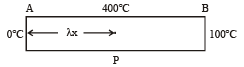For heat flow from P to 0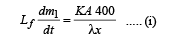For heat flow from P to B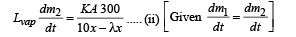On solving (i) and (ii), we get  λ = 9

Q.2. A piece of ice (heat capacity = 2100 J kg–1 °C–1 and latent heat = 3.36 × 105 J kg–1) of mass m grams is at –5°C at atmospheric pressure. It is given 420 J of heat so that the ice starts melting.Finally when the ice-water mixture is in equilibrium, it is found that 1 gm of ice has melted. Assuming there is no other heat exchange in the process, the value of m is

Ans. 8

Solution. Heat supplied = Heat used in converting m grams of ice from –5°C to 0°C + Heat used in converting 1 gram of ice at 0°C to water at 0°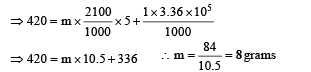Q.3. Two spherical bodies A (radius 6 cm ) and B(radius 18 cm ) are at temperature T1 and T2, respectively. The maximum intensity in the emission spectrum of A is at 500 nm and in that of B is at 1500 nm. Considering them to be black bodies, what will be the ratio of the rate of total energy radiated by A to that of B?

Ans. 9

Solution.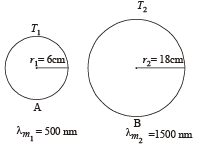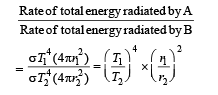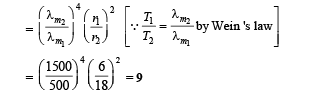Q.4. A diatomic ideal gas is compressed adiabatically to 1/32  of its initial volume. If the initial temperature of the gas is Ti (in Kelvin) and the final temperature is a Ti, the value of a is

Ans. 4

Solution. For an adiabatic process, the  temperature-volume relationship is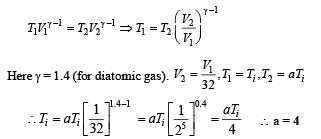Q.5. Steel wire of lenght ‘L’ at 40°C is suspended from the ceiling and then a mass ‘m’ is hung from its free end. The wire is cooled down from 40°C to 30°C to regain its original length ‘L’. The coefficient of linear thermal expansion of the steel is 10–5 /° C, Young’s modulus of steel is 1011 N/m2 and radius of the wire is 1 mm. Assume that L >>diameter of the wire. Then the value of ‘m’ in kg is nearly

Ans. 3

Solution. We know that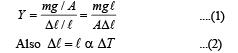From (1) and (2)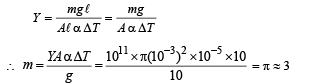Q.6.A thermodynamic system is taken from an initial state i with internal energy Ui = 100 J to the final state f along two different paths iaf and ibf, as schematically shown in the figure. The work done by the system along the paths af, ib and bf are Waf = 200 J, Wib = 50 J and Wbf =100 J respectively. The heat supplied to the system along the path iaf, ib and bf are Qiaf, Qib and Qbf respectively. If the internal energy of the system in the state b is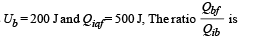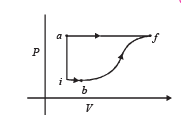Ans. 2

Solution. Applying first law of thermodynamics to path iaf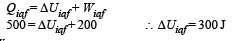Now,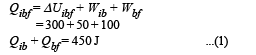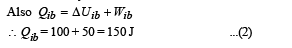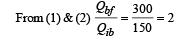Q.7. Two spherical stars A and B emit blackbody radiation. The radius of A is 400 times that of B and A emits 104 times the power emitted from B. The ratio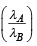of their wavelengths λA and  λB at which the peaks occur in their respective radiation curves is

Ans.2

Solution.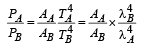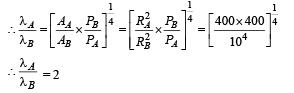Q.8. A metal is heated in a furnace where a sensor is kept above the metal surface to read the power radiated (P) by the metal.The sensor has a scale that displays log2, (P/P0 ), where P0 is a constant. When the metal surface is at a temperature of 487°C, the sensor shows a value 1. Assume that the emissivity of the metallic surface remains constant. What is the value displayed by the sensor when the temperature of the metal surface is raised to 2767°C?

Ans. 9

Solution.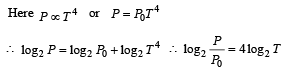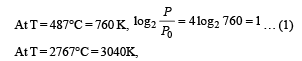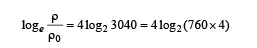=[4 log 2 760+ log22 ]

= 4log2 760 + 8= 1+ 8= 9

The document Integer Answer Type Questions: Heat & Thermodynamics | JEE Advanced Notes | Study Physics 35 Years JEE Main & Advanced Past year Papers - JEE is a part of the JEE Course Physics 35 Years JEE Main & Advanced Past year Papers.
All you need of JEE at this link: JEEUse Code STAYHOME200 and get INR 200 additional OFF Use Coupon Code

Top Courses for JEE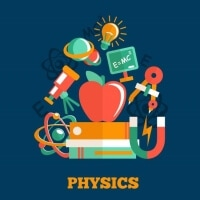Physics 35 Years JEE Main & Advanced Past year Papers

88 docs|49 tests

Top Courses for JEETrack your progress, build streaks, highlight & save important lessons and more!

,

,

,

,

,

,

,

,

,

,

,

,

,

,

,

,

,

,

,

,

,

;